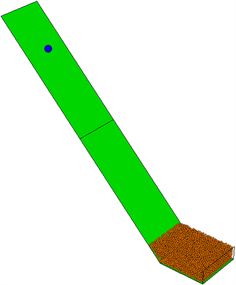﻿ 基于离散元颗粒流的落石冲击力计算公式研究

# 基于离散元颗粒流的落石冲击力计算公式研究Study on the Calculation Formula of Rockfall Impact Force Based on Discrete Element Particle Flow

Abstract: Based on simulation of the rock-falling impact process with the discrete-element particle flow software PFC3D and the simulation calculation, the data of rock-falling impact force is extracted, and analyzed in SPSS software and finally the multi-element linear formula for the impact force of the falling stone is fitted. The method of discrete-element particle flow has great contribution to study of the rockfall impact force and is of very important guidance and reference for the prevention and control of the rockfall disaster in the mountainous area of China.

1. 引言

2. 落石冲击模型介绍

2.1. 落石冲击结构顶板的离散元模型Figure 1. Calculation model of rockfall impact

2.2. 计算工况如表1所示Table 1. Combination of working conditions  

2.3. 离散元数值模拟微观参数设定Table 2. Micro-properties of the model element  

2.4. 离散元数值模拟微观参数标定Table 3. Macro-parameter calibration results of backfill  

3. 多元线性回归理论

3.1. 多元线性回归的定义和模型

$P={B}_{0}+{B}_{1}{X}_{1}+{B}_{2}{X}_{2}+\cdots +{B}_{n}{X}_{n}$ (3-1)

3.2. 线性回归数据处理

1) 建立多元线性回归模型；

2) 求解各个自变量的偏回归系数Bi

3) 回归模型检验，包括相关性检验、相关系数R、R2检验、回归系数t检验，显著性F值检验等等   。

4. 落石冲击力线性回归

4.1. 回归模型分析Table 4. Regression model analysis  

4.2. 方差分析Table 5. Anova analysis of regression model 

4.3. 回归系数显著性分析

4.4. 回归系数相关性检验

4.5. 落石冲击力回归方程

Table 6. Significance analysis and correlation test of regression coefficient

$\begin{array}{l}P=5.983{X}_{1}+7.725{X}_{2}+234.285{X}_{3}-373.307{X}_{4}+0.122{X}_{1}{X}_{2}+2.526{X}_{1}{X}_{3}-4.052{X}_{1}{X}_{4}\\ \text{\hspace{0.17em}}\text{\hspace{0.17em}}\text{\hspace{0.17em}}\text{\hspace{0.17em}}\text{\hspace{0.17em}}+3.677{X}_{2}{X}_{3}-4.117{X}_{2}{X}_{4}-133.48{X}_{3}{X}_{4}+0.055{X}_{1}{X}_{2}{X}_{3}+211.651\end{array}$

$\begin{array}{l}P冲=5.983H+7.725\theta +234.285W-373.307t+0.122H+2.526HW-4.052Ht\\ \text{\hspace{0.17em}}\text{\hspace{0.17em}}\text{\hspace{0.17em}}\text{\hspace{0.17em}}\text{\hspace{0.17em}}\text{\hspace{0.17em}}\text{\hspace{0.17em}}\text{\hspace{0.17em}}+3.677\theta W-4.117\theta t-133.48Wt+0.055H\theta W+211.651\end{array}$

5. 结论

1) 自变量H、W、θ、t对落石冲击力的影响都是显著的；这个结论与文献   的结论是相一致的；

2) 四个自变量一次项、二次交互项对落石冲击力影响显著，个别三次交互项显著，充分说明各个自变量在对落石冲击力影响显著的同时，还对落石冲击力存在交互影响作用，交互项在落石冲击力计算公式研究过程中要予以考虑，不可以忽略不计。

 铁道第二勘测设计院. 铁路工程设计技术手册隧道[M]. 第2版. 北京: 中国铁道出版社, 1995.

 交通部第二公路勘察设计研究院. 公路路基设计规范JTJ013-95[S]. 北京: 人民交通出版社, 1995.

 杨其新, 关宝树. 落石冲击力计算方法的试验研究[J]. 铁道学报, 1996, 18(1): 101-106.

 Kawahara, S. and Muro, T. (2006) Effects of Dry Density and Thickness of Sandy Soil on Impact Response Due to Rockfall. Journal of Terramechanics, 43, 329-340.
https://doi.org/10.1016/j.jterra.2005.05.009

 Labiouse, V., Descoeudres, F. and Montani, S. (1996) Experimental Study of Rock Sheds Impacted by Rock Blocks. Structural Engineering International, 3, 171-175.
https://doi.org/10.2749/101686696780495536

 裴向军, 刘洋, 王东坡. 滚石冲击棚洞砂土垫层耗能缓冲机理研究[J]. 四川大学学报, 2016, 48(1): 15-22.

 杨建荣, 白羽, 杨晓东, 等. 柔性棚洞结构落石冲击数值模拟与试验研究[J]. 振动与冲击, 2017, 36(9): 172-178.

 王静峰, 赵鹏, 袁松, 等. 复合垫层钢棚洞抵抗落石冲击性能研究[J]. 土木工程学报, 2018(S2): 7-13.

 吴佳楠. 钢棚洞结构EPS-细砂复合缓冲层性能[D]: [硕士学位论文]. 成都: 西南交通大学, 2018.

 王永东, 周天跃, 柴伦磊. 基于能量分析的落石对棚洞垫层冲击力学研究[J]. 地下空间与工程学报, 2018, 14(z1): 148-153.

 闫帅星, 何思明, 李新坡. 滚石冲击作用下钢筋混凝土棚洞板动力学响应及冲切[J]. 兰州大学学报, 2019, 55(241): 64-72.

 王玉锁, 李俊杰, 李正辉, 冯高飞, 吴浩, 何俊男. 落石冲击力评定的离散元颗粒流数值模拟[J]. 西南交通大学学报, 2016, 51(1): 22-29.

 李俊杰. 落石冲击力及冲击效应评定的离散元颗粒流数值模拟研究[D]: [硕士学位论文]. 成都: 西南交通大学, 2016.

 杜强, 贾丽艳. SPSS统计分析从入门到精通[M]. 北京: 人民邮电出版社, 2009: 150-256.

Top